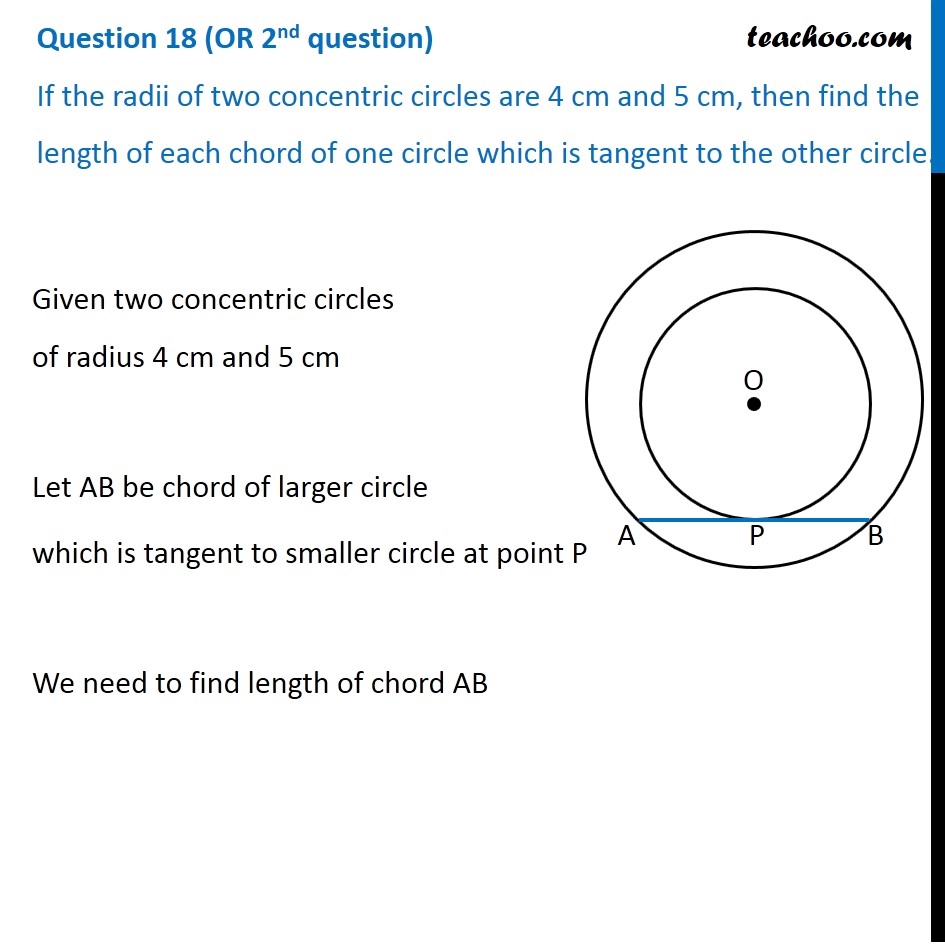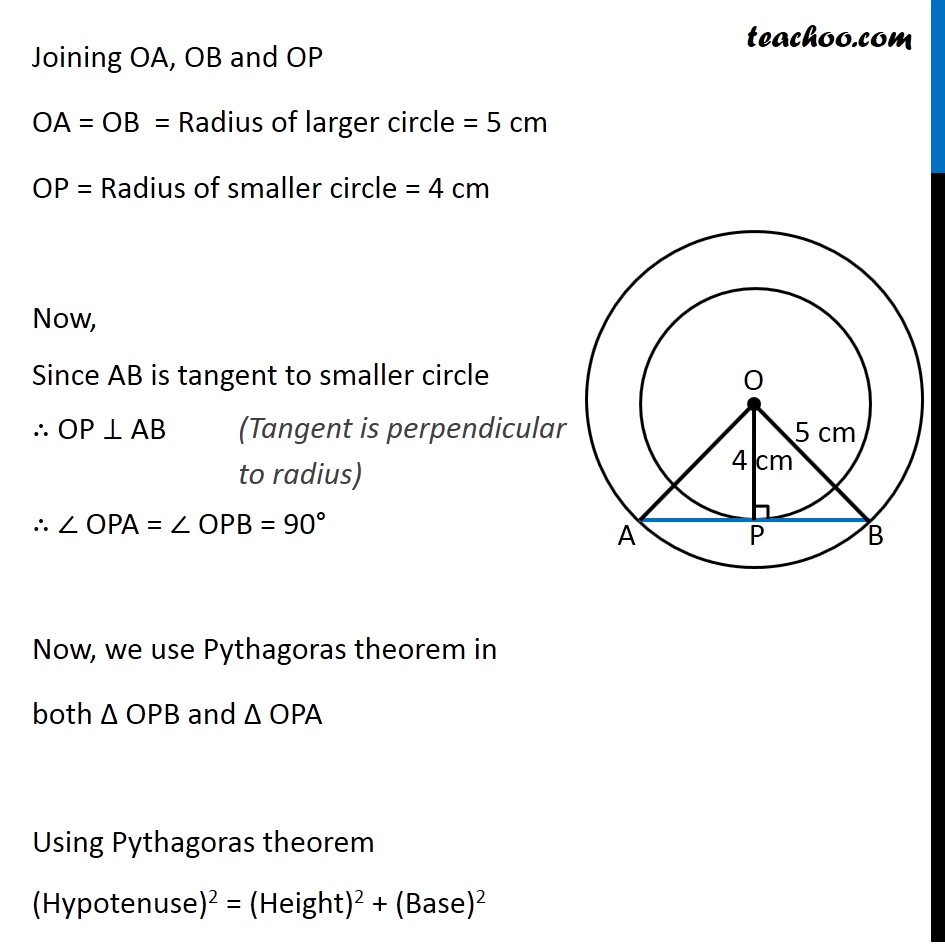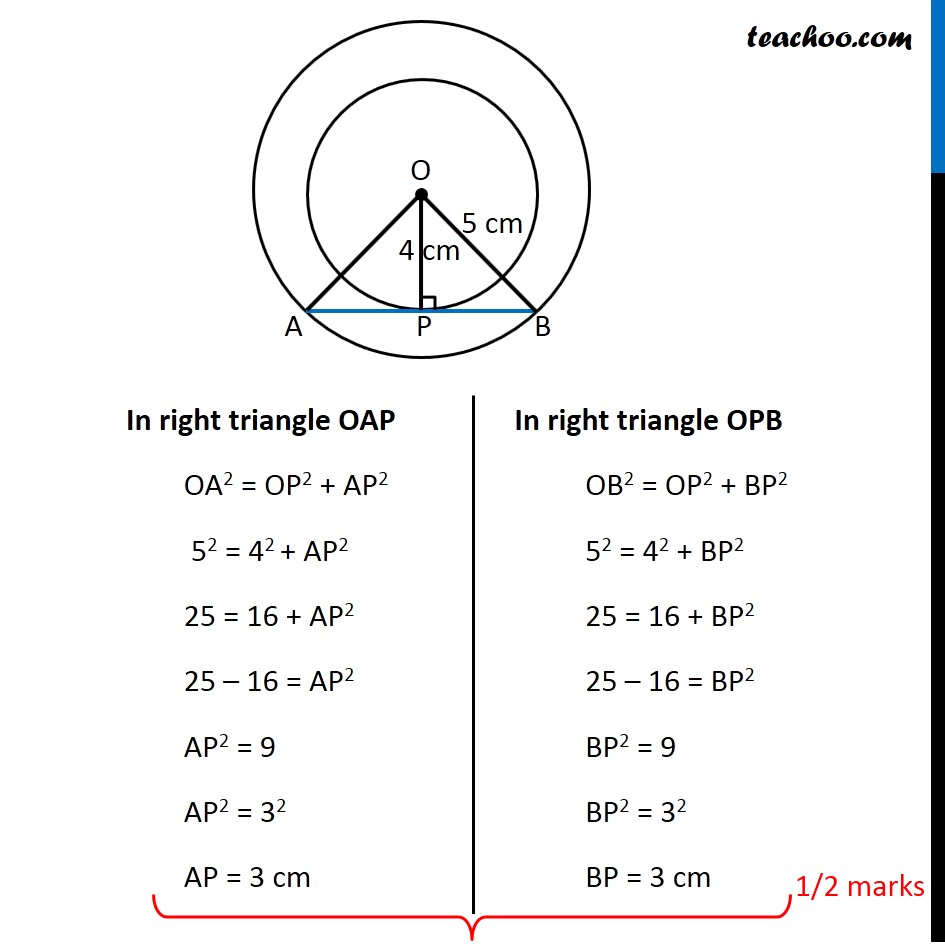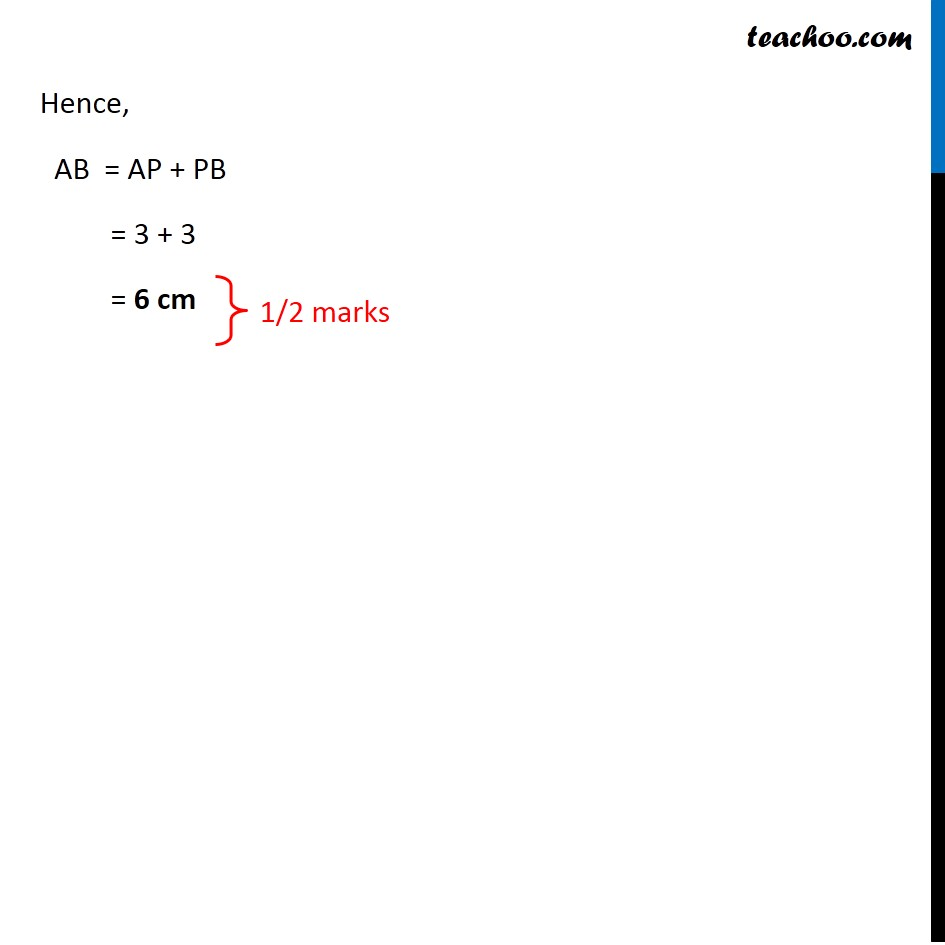CBSE Class 10 Sample Paper for 2020 Boards - Maths Standard

Class 10
Solutions of Sample Papers for Class 10 Boards

## If the radii of two concentric circles are 4 cm and 5 cm, then find the length of each chord of one circle which is tangent to the other circle.Note : This is similar to Ex 10.2, 7 of NCERT – Chapter 10 Class 10

Get live Maths 1-on-1 Classs - Class 6 to 12

### Transcript

Question 18 (OR 2nd question) If the radii of two concentric circles are 4 cm and 5 cm, then find the length of each chord of one circle which is tangent to the other circle. Given two concentric circles of radius 4 cm and 5 cm Let AB be chord of larger circle which is tangent to smaller circle at point P We need to find length of chord AB Joining OA, OB and OP OA = OB = Radius of larger circle = 5 cm OP = Radius of smaller circle = 4 cm Now, Since AB is tangent to smaller circle ∴ OP ⊥ AB ∴ ∠ OPA = ∠ OPB = 90° Now, we use Pythagoras theorem in both Δ OPB and Δ OPA Using Pythagoras theorem (Hypotenuse)2 = (Height)2 + (Base)2 In right triangle OAP OA2 = OP2 + AP2 52 = 42 + AP2 25 = 16 + AP2 25 – 16 = AP2 AP2 = 9 AP2 = 32 AP = 3 cm In right triangle OPB OB2 = OP2 + BP2 52 = 42 + BP2 25 = 16 + BP2 25 – 16 = BP2 BP2 = 9 BP2 = 32 BP = 3 cm Hence, AB = AP + PB = 3 + 3 = 6 cm